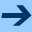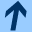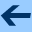NBAC Tutorial

# NBAC Tutorial

## A tiny example

Let us describe a very simle (and maybe stupid) example. We would like to analyse the system depicted on the following figure, where two counters are alternatively incremented.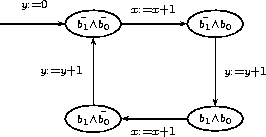This small system is described as follows:

```
state
b0,b1: bool;
x,y : int;

transition
b0' = not b1;
b1' = b0;
b2' = b2 xor (b0 and b1);
x' = if (b0 = b1) then x+1 else x;
y' = if (b0 <> b1) then y+1 else y;

initial not b0 and not b1 and x=0 and y=0;
```

Here we have state variables, but no input variables, as there is no interaction with an environment. Suppose now we want to check that `x` is always greater than or equal to `y`. We add the declaration

```
final not (x>=y);
```

Actually, for some reasons we will explain later, we prefer to "delay" the initial and final sets of states, using a Boolean variable as follows. This gives the program:

```
state
init,ok : bool;
b0,b1: bool;
x,y : int;

transition
init' = false;
ok' = if init then true else    ok and x>=y;
b0' = if init then false else   not b1;
b1' = if init then false else   b0;
x' = if init then 0 else        if (b0 = b1) then x+1 else x;
y' = if init then 0 else        if (b0 <> b1) then y+1 else y;
initial init;
final not init and not ok;
```

Our system corresponds now to the automaton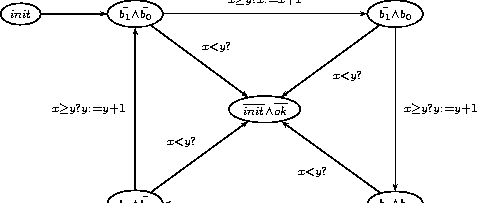## Slicing/verification of the tiny example

Let now ask the tool to perform a step of slicing analysis with the command
nbacg example1.ba -analysis 2 -print "+p +a +r"
The option -analysis 2 indicates we want to perform slicing or verification, the option -print "+p +a +r" indicates we want to have information about initial control analysis (+a) and refinement (+r).

### Preprocessing and creation of the initial control structure

```
- Syntaxical analysis
- Transformation to BDDs
- Treatement of definition of  - Treatement of equation of ok init y x b1 b0
- Treatement of formula: assertion initial final
- Simplification by careset
Variable reordering done
Variable reordering done
- Simplification by Boolean slicing (reach/coreach)
Variable reordering done
Forward analysis (.....)
Backward analysis (..)
Variable reordering done
[ v = 0, etiq =  control=!ok^!init; def=(true, 1>=0); cube=true ,
succ = [],
pred = ,
v = 1, etiq =  control=init; def=(true, 1>=0); cube=true ,
succ = ,
pred = [],
v = 2, etiq =  control=ok^!init; def=(true, 1>=0); cube=true ,
succ = [0; 2],
pred = [1; 2],
]
[ (1,2), bassert=true;
cube=true;
dec=(0.00 prod, 0.00 ite, 1.00 inter, 1.00 dec) ;
(2,0), bassert=y>=x+1;
cube=y>=x+1;
dec=(0.00 prod, 3.00 ite, 1.00 inter, 4.00 dec) ;
(2,2), bassert=!y>=x+1;
cube=!y>=x+1;
dec=(0.00 prod, 3.00 ite, 1.00 inter, 4.00 dec) ;
]
(3 vertices, 3 edges, 1.00 e/v, dev(succ/v) = 0.82 dev(pred/v) = 0.82
postpre : (0.00 prod, 2.00 ite, 1.00 inter, 3.00 dec))
```

First of all, NBAC parses the input file and transform all the expressions into BDDs and MTBDDs. It simplifies the formula by taking into account a careset, which represent the knowledge that some numerical constraints implies other ones. Typically, the fact that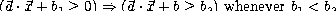is taken into account in the careset.

Then a Boolean slicing is performed: here only the behaviour of Boolean state variables is analysed. Numerical constraints of the involved formula are just viewed as Boolean inputs however constrained by the careset. A partial knowledge about the numerical domain is then taken into account, but in static way: there is no analysis of the dynamic behaviour of numerical variables during an execution at that step.

At last, NBAC build the initial control structure displayed above, with its graphical representation below.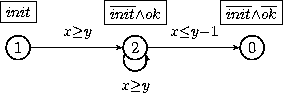The numbers inside the circles are the indices of locations given in NBAC output. Circles define the locations of the control structure. Boxes attached to locations are the definitions of the locations: they define the considered states at a given location. Formulae attached to edges are the assertions of the transition of the control structure: they define necessary conditions for a given transition to be taken during an execution.

The control structure here is generated by the tool by exactly partitioning the current state space (after Boolean slicing) into initial, final, and other states, and then using a (set of) location(s) to represent each equivalence class. The advantage of using two Boolean variables init and final is to ensure that one location is enough to excatly the set of initial states. Otherwise, we have to decompose these sets in an exact union of abstract values.

### First forward/backward analysis cycle

Now NBAC performs a first reachability analysis:

```
*** Analysis ***
[ v = 0, etiq =  control=!ok^!init; def=(true, 1>=0); cube=true ,
succ = [],
pred = ,
v = 1, etiq =  control=init; def=(true, 1>=0); cube=true ,
succ = ,
pred = [],
v = 2, etiq =  control=ok^!init; def=(true, 1>=0); cube=true ,
succ = [0; 2],
pred = [1; 2],                                                           ]
[ (1,2), bassert=true;
cube=true;
dec=(0.00 prod, 0.00 ite, 1.00 inter, 1.00 dec) ;
(2,0), bassert=y>=x+1;
cube=y>=x+1;
dec=(0.00 prod, 3.00 ite, 1.00 inter, 4.00 dec) ;
(2,2), bassert=!y>=x+1;
cube=!y>=x+1;
dec=(0.00 prod, 3.00 ite, 1.00 inter, 4.00 dec) ;                    ]
(3 vertices, 3 edges, 1.00 e/v, dev(succ/v) = 0.82 dev(pred/v) = 0.82
postpre : (0.00 prod, 2.00 ite, 1.00 inter, 3.00 dec))
*** End of analysis in 5 iterations ***
*** narrowing ***
*** End narrowing in 1 iterations without stabilization ***
*** Analysis ***
[ v = 0, etiq =  control=!ok^!init; def=(true, y>=x,y<=x+2,y+x>=2);
cube=true ,
succ = ,
pred = [],
v = 1, etiq =  control=init; def=(true, 1>=0); cube=true ,
succ = [],
pred = ,
v = 2, etiq =  control=ok^!init; def=(true, y>=0,1>=0,y<=x+1,x>=0);
cube=true ,
succ = [1; 2],
pred = [0; 2],
]
[ (0,2), bassert=y>=x+1;
cube=y>=x+1;
dec=(0.00 prod, 3.00 ite, 1.00 inter, 4.00 dec) ;
(2,1), bassert=true;
cube=true;
dec=(0.00 prod, 0.00 ite, 1.00 inter, 1.00 dec) ;
(2,2), bassert=!y>=x+1;
cube=!y>=x+1;
dec=(0.00 prod, 3.00 ite, 1.00 inter, 4.00 dec) ;
]
(3 vertices, 3 edges, 1.00 e/v, dev(succ/v) = 0.82 dev(pred/v) = 0.82
postpre : (0.00 prod, 2.00 ite, 1.00 inter, 3.00 dec))
```

The initial control structure is displayed once again. After end of analysis and narrowing, the resulting control structure is displayed, however in a transposed way (it is made ready for a backward analysis). Let us depict in graphically in the right orientation: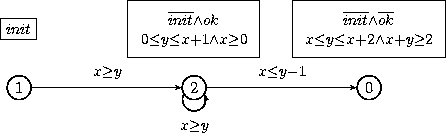What happened ? All the non-rechable states has been removed from the control structure (more precisely, from the definitions). No new information is available on assertions. Let us perform a backward analysis:

```
*** End of analysis in 4 iterations ***
*** narrowing ***
*** End narrowing in 1 iterations with stabilization ***
*** Refinement ***
[ v = 0, etiq =  control=!ok^!init; def=(true, y>=x,y<=x+2,y+x>=2);
cube=true ,
succ = [],
pred = ,
v = 1, etiq =  control=init; def=(true, 1>=0); cube=true ,
succ = ,
pred = [],
v = 2, etiq =  control=ok^!init; def=(true, y<=x+1,1>=0,x>=0,y>=0);
cube=true ,
succ = [0; 2],
pred = [1; 2],
]
[ (1,2), bassert=true;
cube=true;
dec=(0.00 prod, 0.00 ite, 1.00 inter, 1.00 dec) ;
(2,0), bassert=y>=x+1;
cube=y>=x+1;
dec=(0.00 prod, 3.00 ite, 1.00 inter, 4.00 dec) ;
(2,2), bassert=!y>=x+1;
cube=!y>=x+1;
dec=(0.00 prod, 3.00 ite, 1.00 inter, 4.00 dec) ;
]
(3 vertices, 3 edges, 1.00 e/v, dev(succ/v) = 0.82 dev(pred/v) = 0.82
postpre : (0.00 prod, 2.00 ite, 1.00 inter, 3.00 dec))
```

The analysis didn't bring us new information, so the control structure remains unchanged. So a refinement is needed, in order to improve the precision of the analysis.

### Refinement

```
cfc=[[]; ; ; ]
refine_cfc forward
vertex 2, mset=[(b1,1); (b0,1); (y>=x+1,3)]
vertex 0, mset=[]
result = [2 => y>=x+1;]

*** Analysis ***
[ v = 0, etiq =  control=!ok^!init; def=(true, y>=x,y<=x+2,y+x>=2);
cube=true ,
succ = [],
pred = ,
v = 1, etiq =  control=init; def=(true, 1>=0); cube=true ,
succ = ,
pred = [],
v = 2, etiq =  control=ok^!init; def=(true, y=x+1,1>=0,y>=1);
cube=y>=x+1 ,
succ = ,
pred = ,
v = 3, etiq =  control=ok^!init; def=(true, y<=x,1>=0,y>=0);
cube=!y>=x+1 ,
succ = [2; 3],
pred = [1; 3],
]
[ (1,3), bassert=true;
cube=true;
dec=(0.00 prod, 0.00 ite, 1.00 inter, 1.00 dec) ;
(2,0), bassert=true;
cube=true;
dec=(0.00 prod, 3.00 ite, 1.00 inter, 4.00 dec) ;
(3,2), bassert=!b1^b0 + b1^!b0;
cube=true;
dec=(0.00 prod, 0.00 ite, 1.00 inter, 1.00 dec) ;
(3,3), bassert=true;
cube=true;
dec=(0.00 prod, 3.00 ite, 1.00 inter, 4.00 dec) ;                    ]
(4 vertices, 4 edges, 1.00 e/v, dev(succ/v) = 0.71 dev(pred/v) = 0.71
postpre : (0.00 prod, 1.50 ite, 1.00 inter, 2.50 dec))
```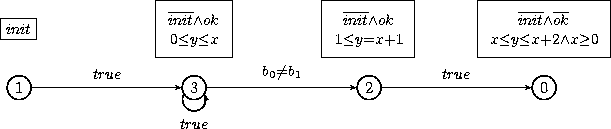The four first lines gives technical information about the refinement heuristic and its results. mset indicates for each condition in the list, how many edges in the control structure would be removed (after a preliminary duplication) if a split of the location is performed w.r.t. this condition. Here NBAC chooses to refine the location 2 w.r.t. the numerical constraint y>=x+1, because it appears to split the greatest number of edges. This gives the new control structure depicted graphically below, where locations 2 and 3 come from the old location 2. Notice that the 3 potential edges (2,0), (3,2), (3,3) doesn't exist in the resulting control structure.

### Second forward/backward analysis cycle

Let us perform a forward analysis:

```
*** End of analysis in 5 iterations ***
*** narrowing ***
*** End narrowing in 1 iterations with stabilization ***
*** Analysis ***
[ v = 0, etiq =  control=!ok^!init; def=(!b1^b0 + b1^!b0, y=x,1>=0,y>=1);
cube=!y>=x+1 ,
succ = ,
pred = [],
v = 1, etiq =  control=init; def=(true, 1>=0); cube=true ,
succ = [],
pred = ,
v = 2, etiq =  control=ok^!init; def=(!b1^!b0 + b1^b0, y=x+1,x>=0,1>=0);
cube=y>=x+1 ,
succ = ,
pred = ,
v = 3, etiq =  control=ok^!init; def=(true, y<=x,1>=0,y>=0);
cube=!y>=x+1 ,
succ = [1; 3],
pred = [2; 3],
]
[ (0,2), bassert=!b1^!b0 + b1^b0;
cube=true;
dec=(0.00 prod, 3.00 ite, 1.00 inter, 4.00 dec) ;
(2,3), bassert=!b1^b0 + b1^!b0;
cube=true;
dec=(0.00 prod, 0.00 ite, 1.00 inter, 1.00 dec) ;
(3,1), bassert=true;
cube=true;
dec=(0.00 prod, 0.00 ite, 1.00 inter, 1.00 dec) ;
(3,3), bassert=true;
cube=true;
dec=(0.00 prod, 3.00 ite, 1.00 inter, 4.00 dec) ;
]
(4 vertices, 4 edges, 1.00 e/v, dev(succ/v) = 0.71 dev(pred/v) = 0.71
postpre : (0.00 prod, 1.50 ite, 1.00 inter, 2.50 dec))
```

which can graphically depicted with: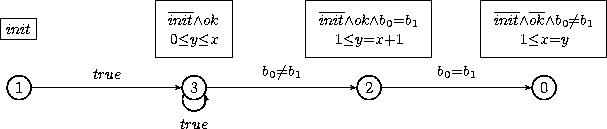We gained new information on locations 2 and 0, and new information on condition attached to edge (2,0). Let us perform a backward analysis:

```
*** End of analysis in 3 iterations ***
*** narrowing ***
*** End narrowing in 1 iterations with stabilization ***
*** Conclusion ***
Success automaton
[ v = 0, etiq =  control=!ok^!init; def=(!b1^b0 + b1^!b0, y=x,1>=0,y>=1);
cube=!y>=x+1 ,
succ = [],
pred = ,
v = 2, etiq =  control=ok^!init; def=(!b1^!b0 + b1^b0, y=x+1,x>=0,1>=0);
cube=y>=x+1 ,
succ = ,
pred = ,
v = 3, etiq =  control=ok^!init; def=(!b1^b0 + b1^!b0, y=x,x>=0,1>=0);
cube=!y>=x+1 ,
succ = [2; 3],
pred = ,
]
[ (2,0), bassert=!b1^!b0 + b1^b0;
cube=true;
dec=(0.00 prod, 3.00 ite, 1.00 inter, 4.00 dec) ;
(3,2), bassert=!b1^b0 + b1^!b0;
cube=true;
dec=(0.00 prod, 0.00 ite, 1.00 inter, 1.00 dec) ;
(3,3), bassert=true;
cube=true;
dec=(0.00 prod, 3.00 ite, 1.00 inter, 4.00 dec) ;
]
(3 vertices, 3 edges, 1.00 e/v, dev(succ/v) = 0.82 dev(pred/v) = 0.00
postpre : (0.00 prod, 2.00 ite, 1.00 inter, 3.00 dec))
```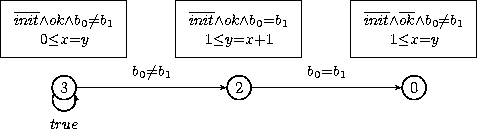The automaton is a "success automaton", because it doesn't contain any more initial locations, which means that initial states cannot lead to a final state. If your goal was to perform slicing, the answer is: "there is no path linking initial and final states". If your goal was verification, the answer is :"the property is true". Here the backward analysis has shown that the edges (3,0) coudn't be taken and that the initial location 1 was not coreachable.

At the end, NBAC displays some statistics:

```
2 cycles of analysis, 1 refinements, 1 divisions
Total time spent in verification : 0.18 sec
Part of it spent in analysis              : 0.14 sec
Part of it spent in ref. heuristics       : 0.01 sec
Part of it spent in partition build & ref : 0.03 sec
Total time spent in post-pre-conditions : 0.09 sec
make      : 0.00 sec;
assertion : 0.02 sec;
apply     : 0.07 sec
SUCCESS: property proved
*** END ***
```

## Generating DOT files

During the refinement process, you can automatically dump the current control structure to a file in DOT format, see graphviz for details.

If we type the command

nbacg example1.ba -analysis 2 -dot "example1.dot +all"

and then convert the DOT file into a Poscript file with the following command (part of graphviz package):

dot -Tps example1.dot >example1.ps

we get the following file example1.ps. With the option +all, the control structure is dump between every analysis step (here, forward analysis followed by a backward analysis). The final automaton being empty in this case, it is not displayed !

## Reachability and coreachability analysis of the tiny example

### Reachability analysis

Let us take the same system, but here we want to perform only reachability analysis. We type the command
nbacg -analysis 0 -o t.ba -print "+a +r -auto 200" example1.ba
The option -analysis 0 means reachability analysis, the option -print "-auto 200" increase the maximal size of control structure which are fully displayed. Last the option -o t.ba indicates that we want to dump the resulting control structure into the file t.ba, in the same format that the format of example1.ba. We get:

```
...

*** Conclusion ***
Valid automaton
[ v = 1, etiq =  control=init; def=(true, 1>=0); cube=true ,
succ = ,
pred = [],
v = 3, etiq =  control=b1^b0^ok^!init; def=(true, y=x,x>=1,1>=0);
cube=!y>=x+1 ,
succ = ,
pred = ,
v = 4, etiq =  control=!b1^b0^ok^!init; def=(true, y+1=x,x>=1,1>=0);
cube=!y>=x+1 ,
succ = ,
pred = ,
v = 5, etiq =  control=b1^!b0^ok^!init; def=(true, y+1=x,x>=2,1>=0);
cube=!y>=x+1 ,
succ = ,
pred = ,
v = 6, etiq =  control=!b1^!b0^ok^!init; def=(true, y=x,1>=0,y>=0);
cube=!y>=x+1 ,
succ = ,
pred = [1; 5],
]
[ (1,6), bassert=true;
cube=true;
dec=(0.00 prod, 0.00 ite, 1.00 inter, 1.00 dec) ;
(3,5), bassert=true;
cube=true;
dec=(0.00 prod, 0.00 ite, 1.00 inter, 1.00 dec) ;
(4,3), bassert=true;
cube=true;
dec=(0.00 prod, 0.00 ite, 1.00 inter, 1.00 dec) ;
(5,6), bassert=true;
cube=true;
dec=(0.00 prod, 0.00 ite, 1.00 inter, 1.00 dec) ;
(6,4), bassert=true;
cube=true;
dec=(0.00 prod, 0.00 ite, 1.00 inter, 1.00 dec) ;
]
(5 vertices, 5 edges, 1.00 e/v, dev(succ/v) = 0.00 dev(pred/v) = 0.63
postpre : (0.00 prod, 0.00 ite, 1.00 inter, 1.00 dec))
3 cycles of analysis, 3 refinements, 4 divisions
Total time spent in verification : 0.26 sec
Part of it spent in analysis              : 0.11 sec
Part of it spent in ref. heuristics       : 0.04 sec
Part of it spent in partition build & ref : 0.11 sec
Total time spent in post-pre-conditions : 0.17 sec
make      : 0.00 sec;
assertion : 0.06 sec;
apply     : 0.11 sec
FAILURE: property not proved
*** OUTPUT ***
Resulting automaton printed to the file t.ba
*** END ***
```

Here the control structure will be refined as long as the heuristics find refinement criteria, then it stops the analysis. There is no verification goal here, so even once final locations are not reachable any more, the process is still continued. The resulting control structure can be graphically depicted: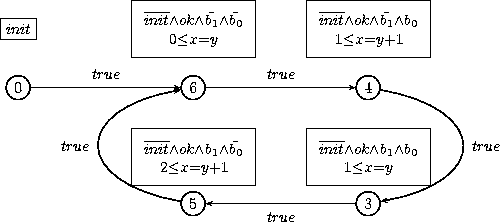### DOT file

If we append the option(s) -dot "example1_reach.dot +all", and we translate to Postscript with dot -Tps example1_reach.dot >example1_reach.ps, we get this.

### Output file

As mentioned above, the resulting control structure, after analysis, can be dumped with the -o t.ba option. Unless the option +success is set and the verification goal is proved, the resulting control structure is dumped to the file t.ba:

```
state
b1, b0, ok, init : bool;
y, x : int;

local
_cond0, _cond2, _cond4, _cond6, _cond8 : bool;
_bdd5, _bdd14, _bdd9, _bdd6, _bdd3, _bdd2, _bdd10, _bdd1, _bdd0, _bdd11,
_bdd12, _bdd7, _bdd4, _bdd13, _bdd8 : bool;
_int14, _int11, _int12, _int0, _int3, _int13, _int8, _int1, _int9, _int5,
_int2, _int6, _int4, _int7, _int10 : int;

definition
_cond0 = b1;
_cond2 = b0;
_cond4 = ok;
_cond6 = init;
_cond8 = y>=x+1;
_bdd5 = if b1 then _bdd0 else _bdd3;
_bdd14 = if b1 then _bdd0 else _bdd12;
_bdd9 = if b0 then _bdd8 else _bdd0;
_bdd6 = if ok then _bdd0 else _bdd3;
_bdd3 = if init then _bdd0 else not _bdd0;
_bdd2 = if init then _bdd0 else not _bdd1;
_bdd10 = if b1 then _bdd9 else _bdd0;
_bdd1 = if _cond8 then _bdd0 else not _bdd0;
_bdd0 = true;
_bdd11 = if b1 then _bdd0 else _bdd9;
_bdd12 = if b0 then _bdd0 else _bdd8;
_bdd7 = if init then _bdd0 else _bdd1;
_bdd4 = if b0 then _bdd3 else _bdd0;
_bdd13 = if b1 then _bdd12 else _bdd0;
_bdd8 = if ok then _bdd7 else _bdd0;
_int14 = if b1 then _int12 else _int13;
_int11 = if init then _int0 else _int10;
_int12 = if b0 then _int9 else _int11;
_int0 = 0;
_int3 = y+1;
_int13 = if b0 then _int11 else _int9;
_int8 = x+1;
_int1 = y;
_int9 = if init then _int0 else _int8;
_int5 = if b0 then _int2 else _int4;
_int2 = if init then _int0 else _int1;
_int6 = if b0 then _int4 else _int2;
_int4 = if init then _int0 else _int3;
_int7 = if b1 then _int5 else _int6;
_int10 = x;

transition
ok' = _bdd2;
init' = not _bdd0;
y' = _int7;
x' = _int14;
b1' = not _bdd4;
b0' = not _bdd5;
assertion _bdd6;
initial _bdd3;
final not _bdd6;
automaton
location init0 : _bdd3;
location good0_n8_b1_b0 : not _bdd10;
location good0_n8_not_b1_b0 : not _bdd11;
location good0_n8_b1_not_b0 : not _bdd13;
location good0_n8_not_b1_not_b0 : not _bdd14;
edge (init0,good0_n8_not_b1_not_b0) : _bdd0;
edge (good0_n8_b1_b0,good0_n8_b1_not_b0) : _bdd0;
edge (good0_n8_not_b1_b0,good0_n8_b1_b0) : _bdd0;
edge (good0_n8_b1_not_b0,good0_n8_not_b1_not_b0) : _bdd0;
edge (good0_n8_not_b1_not_b0,good0_n8_not_b1_b0) : _bdd0;
```

Doesn't look pretty, isn't it ? This is because we want to keep the concise form of the internal BDDs/MTBDDs representation. Each reachable BDD/MTBDD node is declared as a local variable and defined either as a leaf or as a decision node. This textual format can be replace by Disjunctive Normal Forms by using the option -o "-bdd" instead of the default -o "+bdd". Another difference with the initial file is the automaton section, which defines an explicit control structure.

The file t.ba is a valid input to NBAC. For instance, we can type
nbacg -analysis 1 t.ba -o t2.ba
to perform a coreachability analysis after this reachability analysis.

### Controlling the size of the output automaton

There is a way to prevent NBAC to refine too much the control structure. If we type
nbacg -analysis 0 -o t.ba -partition "-maxloc 4" example1.ba
we ask NBAC to stop refinement as soon as the current control structure has at last 4 locations.

### Coreachability analysis of the tiny example

Conversely, if we want to perform a coreachability analysis, which compute the set of states that may lead to a final state, we type the command nbacg -analysis 1 -o t.ba -dot "example1_coreach.dot +all" example1.ba and we obtain this.

Bertrand Jeannet, February 10, 2011NBAC Tutorial